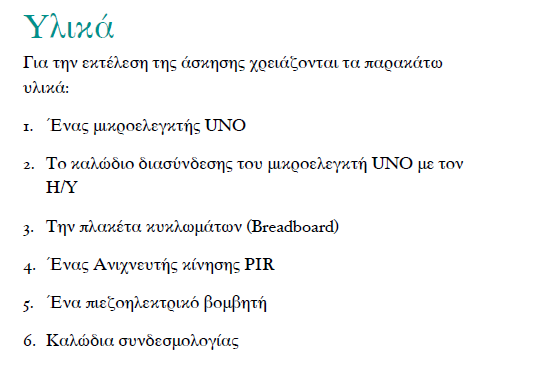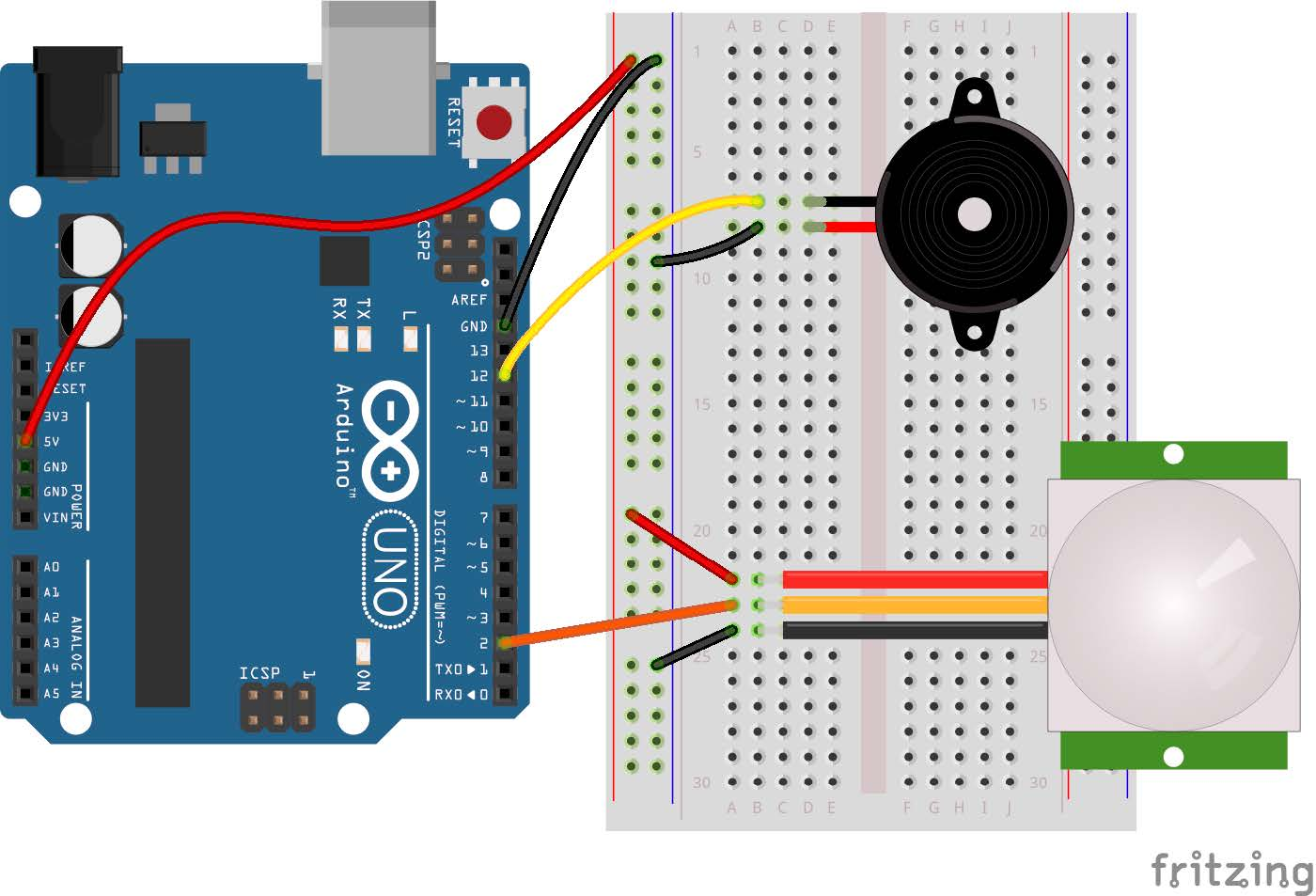tel. 24910 23755 / fax 24910 26620`/*`
`PIR sensor alarm`
`*/`
`// constants won't change. They're used here to`
`// set pin numbers:`
`// in Pin 2 we will connect the PIR sensor`
`// signal. Give it a name.`
`const int pirPin = 2;`
`// in Pin 12 we will connect the piezoelectric`
`// speaker. Give it a name.`
`const int speakerPin = 12;`
`// variables will change:`
`// variable for reading the PIR status`
`// we start, assuming no motion detected`
`int pirState = LOW;`
`// the setup routine runs once when you press`
`// reset:`
`void setup() {`
` // initialize the speaker pin as an output`
` pinMode(speakerPin, OUTPUT);`
` // initialize the PIR pin as an input`
` pinMode(pirPin, INPUT);`
`}`
`// the loop routine runs over and over again`
`// forever:`
`void loop() {`
` // read the state of the PIR sensor`
` pirState = digitalRead(pirPin);`
` // check if the PIR detected motion.`
` // if it is, the pinState is HIGH.`
` if (pirState == HIGH) {`
`   // play siren tones`
`   noTone(speakerPin);`
`   tone(speakerPin, 40, 200);`
`   tone(speakerPin, 494, 500);`
`   tone(speakerPin, 53, 300);`
` } else {`
`   // stop speaker`
`   noTone(speakerPin);`
` }`
`}`

Κατηγορίες: Arduino